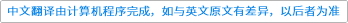The Ramanujan Journal ( IF 0.790 ) Pub Date : 2021-06-10 , DOI: 10.1007/s11139-021-00430-x
Mandeep Kaur, Vandna

Recently, Mc Laughlin proved some results on vanishing coefficients in the series expansions of certain infinite q-products for arithmetic progressions modulo 5, modulo 7 and modulo 11 by grouping the results into several families. In this paper, we prove some new results on vanishing coefficients for arithmetic progressions modulo 7, which are not listed by Mc Laughlin. For example, we prove that if $$t \in \{1,2,3\}$$ and the sequence $$\{A_n\}$$ is defined by $$\sum _{n=0}^{\infty }A_nq^n := (-q^t,-q^{7-t};q^7)_{\infty }(q^{7-2t},q^{7+2t};q^{14})^3_{\infty },$$ then $$A_{7n+4t}=A_{7n+6t^2+4t}=0$$ for all n. Also, we prove four families of results with negative signs for arithmetic progressions modulo 7 classified by Mc Laughlin.down
wechat
bug